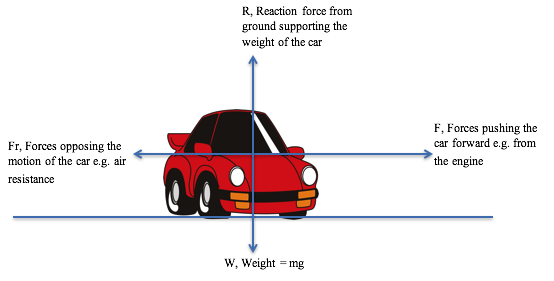# Forces and Motion

Resultant forces and their effects on motionNewtons Second Law of Motion

·      Resultant force is proportional to mass x acceleration

·      In simple terms: Forces cause things to speed up, slow down or change direction·      Force and acceleration are both vectors and taken as positive in the direction of motion

·The previously described Weight force is a special case of this when an object is only subject to acceleration from gravity, the weight, W =mg

In the diagram:

·      The weight and the reaction force are balanced and in opposite directions as the car is on a level road  - hence the resultant vertically is zero

·      The forces pushing the car forward are not equal to the forces opposing the motion of the car – therefore the car must be accelerating toward the right

·      The resultant force toward the right is:     F – Fr

·      The correct use of F=ma is therefore:        F – Fr = ma

Objects Accelerating VerticallyObjects on Slopes

e.g. A box sliding down a slope opposed by friction. If the box has a mass of 2.5kg, the angle of the slope is 30 degrees and it is accelerating at 0.3ms-2, what is the magnitude of the frictional force?

1.     Add all forces onto the diagram with arrows

2.     Split the weight force into components down the slope and perpendicular to the slope – only the component down the slope is important here

= Wsin _ = mg sin _

3.     Find the resultant force down the slope:

= mg sin _ – Fr

4.     Use F=ma

mg sin _ – Fr                                                  = ma

mg sin _ - ma                                                 = Fr

(2.5 x 9.81)sin 30 – (2.5 x 0.3)                   = Fr

11.5N                                                              = Fr

Terminal Velocity

·      For an object in freefall under gravity:

-      It will accelerate to begin with as its weight is larger than the drag of air resistance (Newtons 2nd Law)

-      Its acceleration will decrease over time as the drag becomes larger at higher velocities

-Eventually, the drag force will equal the weight, at this point the forces are balanced and there is no further acceleration – it has reached terminal velocity (Newtons 1st Law)

Vehicle SafetyStopping DistanceCar Safety Features

·      Minimise the force felt by car occupants during a crash

·      They do this using F=ma by reducing the acceleration by increasing the time for the impact

How do the following work?

-      Bumpers

-      Crumple zones

-      Seat belts

-      Airbags

….. any others?